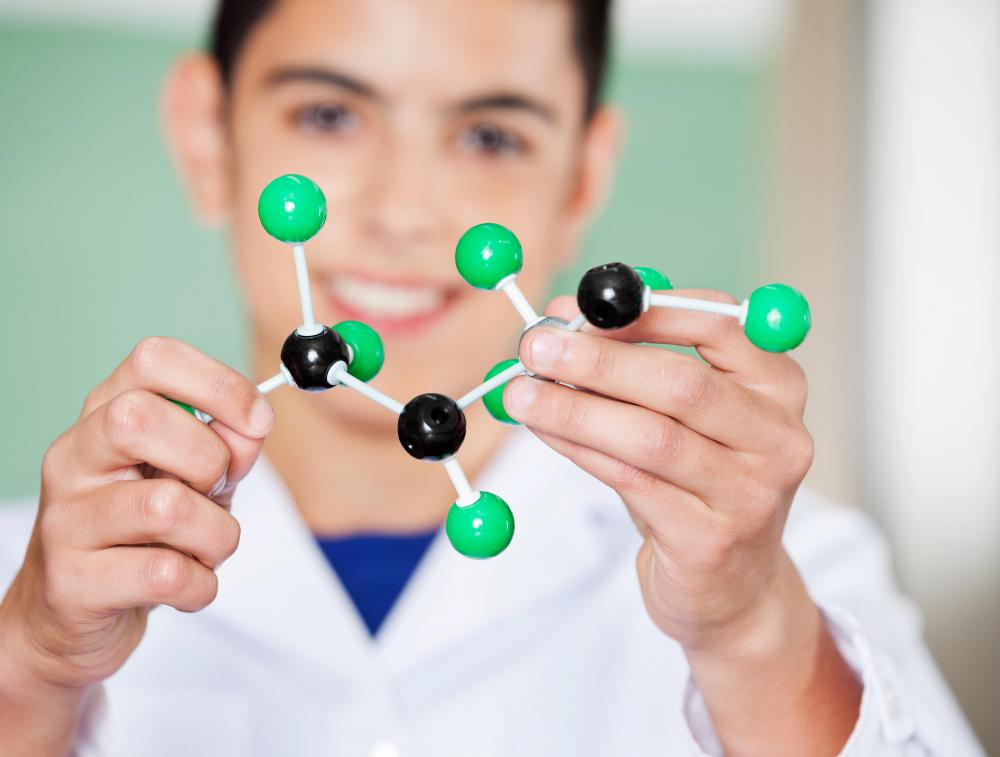# What Is Computational Chemistry?

Jennifer Leigh
Jennifer LeighComputational chemistry utilizes mathematics and computers to solve chemical problems.

Computational chemistry utilizes mathematics and computers to solve chemical problems. By utilizing computer software, chemists can simulate experimental results and find properties of substances. The field of computational chemistry helps to explore things that would otherwise be difficult or costly to find because of the tiny nature of molecules, atoms, and nanoparticles. Much of the field is based upon the Schrodinger equation, which models atoms and molecules using mathematics. Ab initio, semi-empirical, and molecular mechanics are methods of computational chemistry often used to analyze molecular structures.Computational chemistry is often used to analyze molecular structure.

The computational chemistry process begins by looking at a theory, such as the Electronic Structure Theory. This helps to determine the motion of the electrons within a molecule. At this point, using mathematical equations, a basis set can be determined based upon the calculations. This information can be inputted into computer software to describe such things as the wave function, which can be used to create models of other physical characteristics of the molecule. Chemists can see a model of the orbitals of the molecule, begin predicting experimental structures, and look at the energy of the molecule.

Using ab initio allows chemists to look at physical properties of a substance and use the Schrodinger equation to find out physical characteristics of molecules. This includes such things as the geometry of molecules, the dipole moment, and the energy of a reaction. Vibrational frequencies, the reaction rate, and free energy can also be found using ab initio. Since these physical characteristics are extremely difficult to solve, it is necessary for computational chemists to simplify them enough that the physical characteristics can be found and still be accurate.

Molecular mechanics is a method of computational chemistry used in biochemistry experiments and applications. This method can be used for larger structures such as enzymes and relies on traditional physics, but is not able to calculate electronic properties in substances. The field of computational chemistry is constantly changing as technology advances and new theories are developed.

These techniques allow chemists to examine structures that would be nearly impossible to look at otherwise, due to their extremely small size. Nanoparticles, which are smaller than atoms, can be modeled for use in applications such as electronics, explosives, and medicine. Since much of computational chemistry is based upon modeling of known properties, there is room for error in these experiments. This is why advanced training and knowledge in chemistry and research are necessary to work in computational chemistry.

## You might also Like## Discuss this Article

Forgot password?
###### Register:
•Computational chemistry utilizes mathematics and computers to solve chemical problems.
•Computational chemistry is often used to analyze molecular structure.